# How to use the SUMIF function in Excel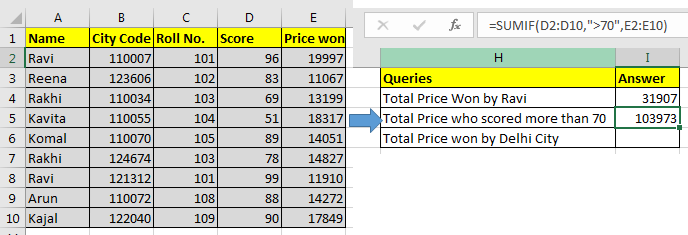In this article, we will learn How to use the SUMIF function in Excel.

What is the SUMIF function ?

In Excel we calculate the sum having given criteria. For Example finding the sum of salaries which are greater than 50k or finding the sum of scores obtained by Jackie in Exams. This is usually a useful function when the given criteria is only one. Criteria range can be the same as sum range or can be different depending upon the situation.

SUMIF Function in Excel

 =SUMIF(condition_range,condition,sum range)

Pro Notes:

• Conditions must be given using the double quotes (">15000").
• If your sum range and condition range are the same, you can omit the sum_range variable in the SUMIF function. =SUMIF(E2:E10,">15000") and =SUMIF(E2:E10,">15000",E2:E10) will produce the same result, 56163.
• Text values are encapsulated in double quotes, but numbers do not. =SUMIF(C2:C10,103,E2:E10) this will work fine and will return 28026. However while working with logical operators you need to use double quotes. Like our example =SUMIF(D2:D10,">70",E2:E10)
• It can check only one condition. For multiple conditions we use the SUMIFS function in Excel.

Example :

All of these might be confusing to understand. Let's understand how to use the function using an example. Here we will try out the SUMIF function. As the name suggests, the SUMIF formula in Excel sums values in a range on a given condition.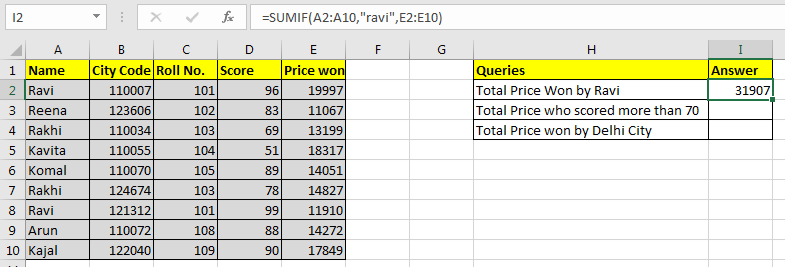Generic Excel SUMIF Formula:

 =SUMIF(condition_range,condition,sum range)

Let's jump into an example. But theory…, Ah! We will cover it later.

#### Use SUMIF To Sum Values On One Condition

For this example I have prepared this data.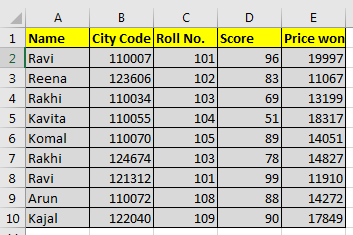Based on this data we need to answer these questions: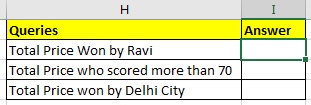#### SUMIF with Text Condition

We need to tell the sum of the price won by Ravi.

So our condition range will be name range and that is A2:A10.

Our condition is Ravi and

Sum range is E2:E10.

So in cell I2 we will write:

 =SUMIF(A2:A10,"ravi",E2:E10)

Note that ravi is in double quotes. Text conditions are always written in double quotes. This is not the case with numbers.

Note that ravi is written in all smalls. Since SUMIF is not case sensitive, hence it doesn’t matter.

The above SUMIF formula will return 31907 as shown in the image below.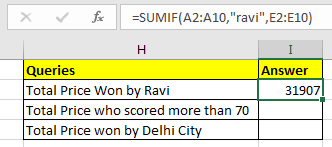#### SUMIF with Logical Operators

For the second question, our condition range will be D2:D10.

Condition is >70 and

The sum range is the same as before.

 =SUMIF(D2:D10,">70",E2:E10)

The above SUMIF formula will return 103973 as shown in the image below.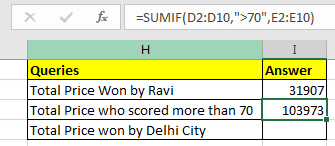#### SUMIF with Wild Card Operators

In the third question, our condition is Delhi. But we don’t have a city column. Hmmm… So what do we have? Aha! City code. This will work.

We know that all Delhi city codes start from 1100. City codes are in 6 digits. So we know it is 1100??. “?” operator is used when we know number of characters but don’t know the character. As here we know that there are two more numbers after 1100. They can be anything, so we used “?”. If didn’t know the number of characters, we would use “*”.

Remember wild card operators only work with text values. Hence you need to convert city code into text.

You can concatenate numbers with “” to make them text value.

 (formula to convert number into text)            = number & “”   or  =CONCATENATE(number,””)

Now in cell I2 write this formula

 =SUMIF(B2:B10,"1100??",E2:E10)

This will return the sum of the price whose city code starts with 1100. In our example it is 79836.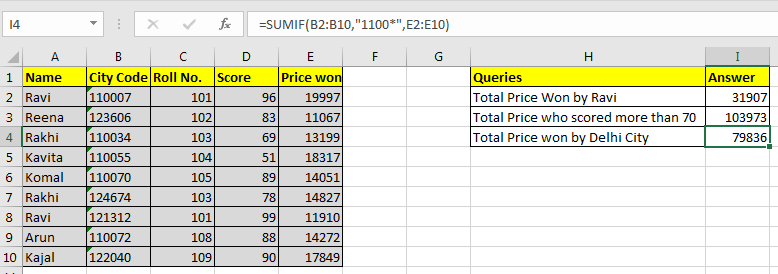Here are all the observational notes when error is given SUMIF function in Excel
Notes :

1. If SUMIF is returning #N/A error or any other error, evaluate the formula. There is 80% chance that you will get your formula working. To evaluate formulas, select the formula cell and go to the Formula tab in ribbon. Here find Evaluate Formula option. You will find it in the Auditing section.
2. If you are writing the correct formula and when you update the sheet, the SUMIF function doesn't return an updated value. It is possible that you have set formula calculation to manual. Press F9 key to recalculate the sheet.
3. Check the format of the values involved in the calculation. It is very likely to have unexpected formats if you have imported data from other sources.

Hope this article about How to use the SUMIF function in Excel is explanatory. Find more articles on calculating values and related Excel formulas here. If you liked our blogs, share it with your friends on Facebook. And also you can follow us on Twitter and Facebook. We would love to hear from you, do let us know how we can improve, complement or innovate our work and make it better for you. Write to us at info@exceltip.com.

Related Articles :

How to use the SUMPRODUCT function in Excel: Returns the SUM after multiplication of values in multiple arrays in excel.

SUM if date is between : Returns the SUM of values between given dates or period in excel.

Sum if date is greater than given date: Returns the SUM of values after the given date or period in excel.

2 Ways to Sum by Month in Excel: Returns the SUM of values within a given specific month in excel.

How to Sum Multiple Columns with Condition: Returns the SUM of values across multiple columns having condition in excel

How to use wildcards in excel : Count cells matching phrases using the wildcards in excel

Popular Articles :

How to use the IF Function in Excel : The IF statement in Excel checks the condition and returns a specific value if the condition is TRUE or returns another specific value if FALSE.

How to use the VLOOKUP Function in Excel : This is one of the most used and popular functions of excel that is used to lookup value from different ranges and sheets.

How to use the SUMIF Function in Excel : This is another dashboard essential function. This helps you sum up values on specific conditions.

How to use the COUNTIF Function in Excel : Count values with conditions using this amazing function. You don't need to filter your data to count specific values. Countif function is essential to prepare your dashboard.

## Users are saying about us...

1.How do I work out in Excel, the cost of work that has taken say, from 9:00 till 10:oo is it £20, then till 11:30 is it £28 and then till 12:45 is it £45

Terms and Conditions of use

The applications/code on this site are distributed as is and without warranties or liability. In no event shall the owner of the copyrights, or the authors of the applications/code be liable for any loss of profit, any problems or any damage resulting from the use or evaluation of the applications/code.